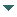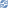# gvSIG

##### Personal tools
You are here: Home Point
gvSIG DesktopgvSIG Desktop

Cached time 11/21/13 11:24:39# Point

Up one level
 ◄ Previous Geometry examples Next: Curve ►

To create a geometry of the Point type, is necessary to use the code Point of the list on TYPES. We can use the generic method from the GeometryManager to create the geonometry, to do a casting to Point and to assign the values. For example, to create a 2-dimensional geometry with the coordinates 2,4 we need to execute the following code:

```Point point = (Point) geometryManager.create(TYPES.POINT, SUBTYPES.GEOM2D);
point.setX(2);
point.setY(4);
```

There're directly methods both to write (setX and setY) and to read (getX and getY) the first and the second coordenate. To edit or recuperate the rest of values from the other dimensions you can use the generic methods setCoordinateAt and getCoordinateAt.

The manager has directly methods to create the most common geometries with the intent that we don't need to do a casting and the assignation of the values to the two first coordinates. In the Point case, we can use the method createPoint from the manager, where we must indicate the subtype of the point and the values of the first and second coordenate.

```Point point = geometryManager.createPoint(2, 4, SUBTYPES.GEOM2D);
```

There aren't specific methods for all the kinds of geometries. For example, to create a 3-dimensional point which has the coordenates 1,1,5 we must execute the following code:

```Point point = geometryManager.createPoint(1, 1, SUBTYPES.GEOM3D);
point.setCoordinateAt(2, 5);
```

Another option could be do it for each coordenate, and the result point will be the same:

```Point point = (Point) geometryManager.create(TYPES.POINT, SUBTYPES.GEOM2D);
point.setX(1);
point.setY(1);
point.setCoordinateAt(2, 5);
```
 ◄ Previous Geometry examples Next: Curve ►